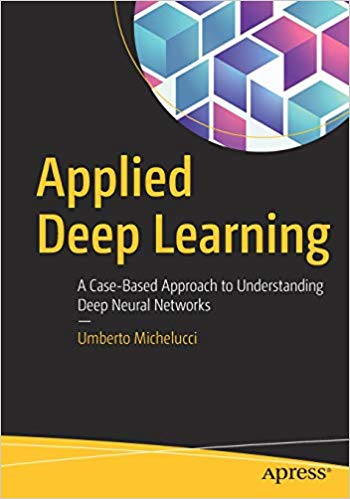# Applied Deep Learning – pdf – 电子书免费下载Work with advanced topics in deep learning, such as optimization algorithms, hyper-parameter tuning, dropout, and error analysis as well as strategies to address typical problems encountered when training deep neural networks. You’ll begin by studying the activation functions mostly with a single neuron (ReLu, sigmoid, and Swish), seeing how to perform linear and logistic regression using TensorFlow, and choosing the right cost function.

The next section talks about more complicated neural network architectures with several layers and neurons and explores the problem of random initialization of weights. An entire chapter is dedicated to a complete overview of neural network error analysis, giving examples of solving problems originating from variance, bias, overfitting, and datasets coming from different distributions.

Applied Deep Learning also discusses how to implement logistic regression completely from scratch without using any Python library except NumPy, to let you appreciate how libraries such as TensorFlow allow quick and efficient experiments. Case studies for each method are included to put into practice all theoretical information. You’ll discover tips and tricks for writing optimized Python code (for example vectorizing loops with NumPy).

What You Will Learn

• Implement advanced techniques in the right way in Python and TensorFlow
• Debug and optimize advanced methods (such as dropout and regularization)
• Carry out error analysis (to realize if one has a bias problem, a variance problem, a data offset problem, and so on)
• Set up a machine learning project focused on deep learning on a complex dataset

Who This Book Is For

Readers with a medium understanding of machine learning, linear algebra, calculus, and basic Python programming.

ISBN:1484237897

## 锦瑟无端五十弦，一弦一柱思华年

### 觉得文章有用就打赏一下文章作者

#### 支付宝扫一扫打赏#### 微信扫一扫打赏